# References and Basics

References:

Definition: A map $$\pi: \Sigma \to \Sigma'$$ of Riemann surfaces is said to be ramified at a point $$p\in \Sigma'$$ iff in local charts $$\pi$$ has the form $$z\mapsto z^n$$ for some $$n>1$$.

I.e. all points in a punctured neighborhood of $$\pi(p)$$ have $$n$$ preimages.

Definition: If $$\pi$$ is ramified at $$p$$, the number of preimages $$n$$ is referred to as $$e_p$$, the ramification index of $$p$$.

Fact: $$\mathbf{\beta}(\Sigma_g) = [1, 2g, 1, 0, \cdots]$$ and $$\chi(\Sigma) = 2-2g$$.

Theorem: If $$\pi$$ is an unramified covering map of degree $$n$$, then $$\chi(\Sigma') = n\chi(\Sigma)$$.

Theorem (Riemann-Hurwitz): If $$\pi: \Sigma \to \Sigma'$$ is a ramified covering map of degree $$N$$, then

\begin{align*} \chi(\Sigma') = N \chi(\Sigma) - \sum (e_p - 1) \quad\text{ i.e. } 2 g(\Sigma') - 2= N(2g(\Sigma) - 2) + \sum (e_p - 1) .\end{align*}

Another useful form: Let $$r \in \Sigma'$$ be the number of ramification points, and $$b$$ the number of branch points, i.e. their images in $$\Sigma$$. Then

\begin{align*} \chi(\Sigma') = N(\chi(\Sigma) - b) + r .\end{align*}

Holomorphic Forms: A holomorphic $$p{\hbox{-}}$$form on $$X$$ is a section of $$\Lambda^p T^\vee X$$, the $$p$$th exterior power of the holomorphic cotangent bundle of $$X$$.

For $$n = \dim_{\mathbb{C}}X$$, the $$n{\hbox{-}}$$forms are an important special case. Any such form $$w$$ is given in local coordinates $$(z_1, \cdots, z_n)$$ by

\begin{align*} w = w(z_1, \cdots, z_n) dz_1 \wedge \cdots \wedge dz_n \end{align*}

for some holomorphic function $$w: {\mathbb{C}}^n \to ?$$.

Canonical Bundle: Given a complex manifold $$M$$, we can define the tangent bundle $${\mathbb{C}}^n \to TM \to M$$ and the cotangent bundle $${\mathbb{C}}^n \to T^\vee M \to M$$, which we’ll just denote $$T^\vee M$$. Then the canonical bundle is the bundle $${\mathbb{C}}\to \Lambda^n T^\vee M \to M?$$, denoted by $$\omega$$, obtained by taking the $$n$$th exterior power.

It is a theorem that the fibers are in fact complex lines $${\mathbb{C}}^1$$. For vector bundles, this is referred to as the determinant bundle. If $$M$$ is a smooth manifold, then $$\omega$$ has a global section.

Note: a holomorphic $$n{\hbox{-}}$$form is exactly the same as a section of the canonical bundle.

Interesting aside: a Calabi-Yau is a manifold with a nowhere vanishing holomorphic $$n{\hbox{-}}$$form, which implies that the canonical bundle admits a map to a trivial line bundle that is an isomorphism, i.e. the canonical bundle is trivial.

Exercise: For $$\Sigma_g$$ a compact Riemann surface of genus $$g$$, the dimension of the space of holomorphic sections of the canonical bundle, i.e. the space of holomorphic differentials on $$\Sigma_G$$, is given by $$\dim H^0(X; \Omega) = g$$ (the genus of the surface). Proof: use Riemann-Roch.

Classification of elliptic orbifolds of dimension 2: Define $$(n_1, \cdots; m_1, \cdots)$$ as the profile, where $$n_i$$ are elliptic points (locally look like quotient by $${\mathbb{Z}}/n{\mathbb{Z}}$$), and $$m_i$$ are corner reflectors (locally look like quotient by a dihedral group):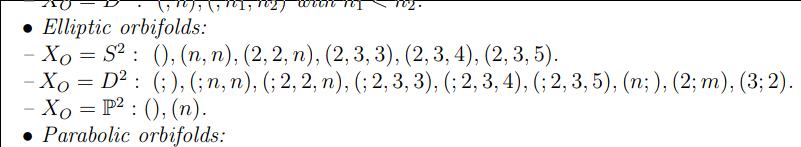Conformal (or equivalently complex) structures on a genus $$g$$ surface form a moduli space $${\mathcal{M}}_g$$ of dimension $$3g-3$$ for $$g > 1$$.

Let $$\alpha$$ be any partition of $$2g-2$$, and $${\mathcal{H}}(\alpha)$$ the moduli space of pairs $$(\Sigma_g, \omega)$$ where $$\Sigma_g$$ is a Riemann surface of genus $$g$$ and $$\omega$$ is a holomorphic 1-form (Abelian differential) on $$M$$ with the orders of its zeros given by $$\alpha$$. Letting $${\mathcal{H}}$$ be the moduli space of all abelian differentials on Riemann surfaces of genus $$g$$ is stratified by $${\mathcal{H}}(\alpha)$$ as $$\alpha$$ ranges over all partitions. For flat tori, $${\mathcal{H}}= \operatorname{GL}_+(2, {\mathbb{R}})/{\text{SL}}(2, {\mathbb{Z}})$$.

For $$\Sigma_g$$ a Riemann surface, there is a formula (Gauss-Bonnet in the flat metric) relating the degrees of the zeros of a holomorphic 1-form to the genus:

\begin{align*} \sum d_j = 2g-2 .\end{align*}

# Notes on Paper

Reference: https://arxiv.org/abs/math/0609392

## Section 1

Flat surfaces are characterized as surfaces with a flat metric and (finitely many?) cone-like singularities. These surfaces appear to be isomorphic to moduli spaces of holomorphic 1-forms. It is profitable to study the orbit of the surface under the Teichmüller geodesic flow, as well as a $$\operatorname{GL}_n$$ action.

Some introductory surveys:

• A. Eskin [E],
• G. Forni [For2],
• P. Hubert and T. Schmidt [HuSdt5]
• H. Masur [Ma7],
• H. Masur and S. Tabachnikov [MaT]
• J. Smillie [S].

We usually associate

• Constant positive curvature = $$S^2$$
• Constant zero curvature = $$S^1 \times S^1 \mathrel{\vcenter{:}}= T^1 = \Sigma_1$$
• Constant negative curvature = $$\Sigma_g$$ for $$g\geq 2$$, a surface of higher genus

Proposition: Any surface can be given a flat metric, possibly introducing singular points.

Idea: Push all of the curvature into a cone point.

Example: The standard cube embedded in $${\mathbb{R}}^3$$.

This is a flat surface with 8 cone points located at the vertices. Note that the metric is non-degenerate on the edges, since any neighborhood of a point on an edge is still homeomorphic to $${\mathbb{R}}^2$$:

Any neighborhood of a vertex is isometric to the vertex of the usual notion of a cone. The cone angle can be measured by cutting a cone from the base to the vertex, yielding a flat pattern that sits in $${\mathbb{R}}_2$$, and measuring the “missing” angle in the resulting circle:

This shows that the cone angle of the cube is $$3\pi/2$$, which coincides with the fact that there are 3 square (and thus 3 right angles) adjacent to any cone point.

Definition: Geodesic (todo)

Definition: Ergodic (todo)

Here this means that a typical geodesic will visit any region in phase space and time spent in a region is proportional to volume.

Definition: Holonomy and Holonomy group (todo)

General (wildly open) problem:

• Describe the behavior of a generic geodesic on a surface
• Prove that the geodesic flow is ergodic on a typical flat surface
• Does almost every surface have a closed geodesic that does not pass through singular points?
• If so, how many?
• Find the (asymptotic) number of such closed geodesics of length shorter than $$L$$

This remains unsolved for $$S^2$$ with 3 singularities (equivalent to a certain billiards problem). It is not even known if any flat sphere admits a single closed geodesic.

Flat surfaces have nontrivial holonomy, which makes them resemble Riemannian manifolds more than flat tori.

If we take the surface and puncture the conical points, it is locally isometric to the punctured Euclidean plane. This allows a notion of parallel transport of tangent vectors.

Parallel transports along homotopically trivial loops are always the identity; otherwise, for homotopically nontrivial loops this rotates the vector by some angle. Parallel transport around a cone point rotates by exactly the cone angle. Nontrivial holonomy forces geodesics to self-intersect many times.

Exercise: parallel transport a vector around the cone point of a cube.

The flat torus has trivial linear holonomy – all geodesics either close up, or never self-intersect and produce a dense winding path.

Definition: A translation surface is closed orientable surface with a flat metric, a finite number of conical singularities, and trivial linear holonomy.

Note that trivial linear holonomy implies that cone angles are all integer multiples of $$2\pi$$.

Convention: we assume all flat surfaces come with a distinguished direction.

Remark: Billiards gives rise to a flat surface with nontrivial linear holonomy.

Definition: A half-translation surface is a surface with a flat metric and holonomy group $${\mathbb{Z}}/(2)$$.

In this case, a vector $$\mathbf{v}$$ may come back as $$-\mathbf{v}$$ after parallel transport.

We’ll often consider families of flat surface sharing the same genus and number/type of conical singularities. These will correspond to strata of moduli spaces of one-forms.

It will often be useful to let $${\text{SL}}(2, {\mathbb{R}})$$ act on these families, consider the orbits, and take its closure.

Central problem / conjecture: Taking the closure of an orbit under the action of $$\operatorname{GL}^+(2, {\mathbb{R}})$$ is a complex subvariety, so both the moduli space of holomorphic one-forms and the moduli space of quadratic differentials resemble homogeneous spaces under the action of a unipotent group.

The there is a projection from these orbits (Teichmüller discs) to the moduli space of complex structures (?), which will be denoted $${\mathcal{M}}_g$$. It is well-known that moduli spaces are not homogeneous spaces, but the conjecture here is that they behave as if they were.

## Section 2: Motivations

Open problems in rectangular billiards:

1. Describe the behavior of a billiard trajectory in a generic triangle, and prove that the billiard flow is ergodic.
2. Does (almost) any table have at least one regular periodic trajectory? Is it preserved under deformations?
3. Asymptotically in length, how many periodic trajectories are there?
4. Does any obtuse triangle have a single periodic trajectory?

Known example: Acute triangles have at least 1, see Fagnano trajectory

Fox-Kershner construction: Yields a way to go from billiard trajectories to geodesics on a flat surface. General idea: glue two copies of the billiard table along the edge to get a flat sphere; then paths lift to geodesics. Such surfaces are not “very flat”, i.e. they have nontrivial linear holonomy.

# Thursday, Week 1

Motivation: Gauss’ Unicursal Problem. How many distinct curves $$\alpha: [0, 1] \to {\mathbb{R}}^2$$ are there with no triple crossings?

Note that if we compactify the plane to the Riemann sphere (and possibly take the curve to be piecewise linear) then we obtain a tiling of the sphere. We can also take a dual tiling by taking the barycenters of each polygon and connecting them by an edge iff their corresponding polygons share an edge.

Definition: A translation surface is the 2-dimensional topological manifold obtained by taking any set of polygons in $${\mathbb{R}}^2$$ and gluing their edges by translations.

Example: Any elliptic curve (topologically a torus) is a translation surface.

We take equivalence up to cutting, pasting, and rearranging.

Lemma: Two elliptic curves (of genus 2) are isomorphic iff the translation surfaces differ by a homothety, i.e. a rotation and scaling.

Note: we will eventually see that the data of a translation surface is equivalently a holomorphic 1-form.

Definition: A half-translation surface is a translation surface where we now additionally allow gluing by rotations of $$\pi$$ radians.

Example:

Note that the gluing $$b$$ now requires a rotation.

By gluing edges with matching letters, we get a “hot pocket” surface:

Lemma: Any game of rectangular billiards yields a half-translation surface.

Given any domain for rectangular billiards, we inflate it to a surface:

We can then cut along everything but the bottom edge to “unwrap” it into a half-translation surface:

Some questions related to rectangular billiards:

Question 1: Given a random starting point and direction, what proportion of the total region is traversed? Will the trajectory entire a given region? How long does the billiard spend in any given region?

Theorem: The percentage of time spent in a given region is equal to the proportion of its area to the total area.

This requires some ergodic theory.

Question 2: If you shine a laser from a given spot, is the entire region illuminated?

Theorem: No! There is a counterexample with 18 sides. Moreover, no positive-area region can be avoided, but certain finite sets can.

Definition: A flat surface is a generalization of translation surfaces that now allows gluing by any isometry of $${\mathbb{R}}^2$$.

Example: A cube in $${\mathbb{R}}^3$$ is a flat surface, noting that the planar gluing diagram for it now requires rotations of $$\pi/2$$ radians: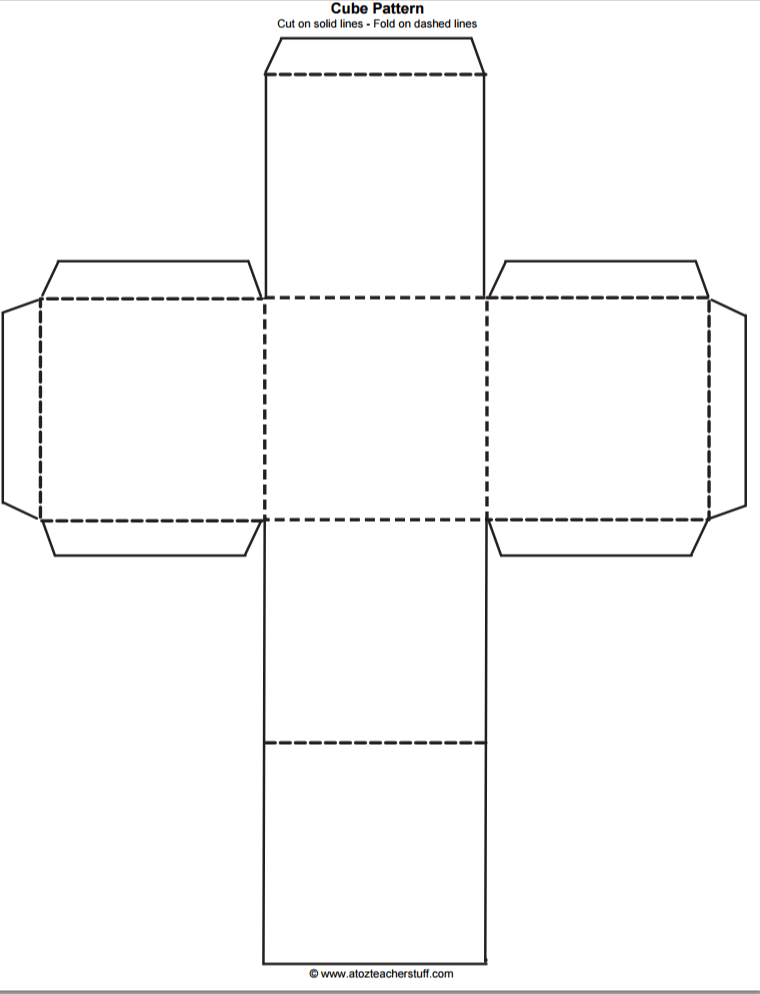Note that we can form higher genus surfaces using polygon gluing:

Exercise: Check that there is only one vertex in this polygon.

In general, the vertices may have total angle greater than $$2\pi n$$. We refer to these as cone points, and the total angle as the cone angle.

Exercise: Check that the cone angle in the above example is $$6\pi$$ by taking a loop around the cone point.

Note on a weird phenomenon: it seems difficult to find $${\mathbb{Z}}^2$$ or $${\mathbb{Q}}^2$$ points on the sloped edges of a regular polygon, based on computer drawings.

Remark: This flat surface admits charts to $${\mathbb{C}}$$ with the following transition functions. Let $$P$$ denote the single cone point.

Then there is a 3-fold cover give by the following space, thought of as a singular helical

which maps onto the unit disc in $${\mathbb{C}}$$:

The covering map from the former to the latter is given by $$z\mapsto z^{\frac 1 3}$$, which coincides with the fact that the cone angle at $$P$$ is $$3(2\pi) = 6\pi$$.

One can also imagine this space in $${\mathbb{R}}^3$$ with a projection onto the plane:

Here the helicoid goes through three full twists, where the top and bottom pieces are identified.

Proposition: In a neighborhood of a cone point $$P$$ with cone angle $$2\pi n$$, the map $$z\mapsto z^{\frac 1 n}$$ will be a local chart for any $$z$$ in a neighborhood of $$P$$.

This gives the resulting surface the structure of a Riemann surface, i.e. a surface admitting charts to $${\mathbb{C}}$$ with holomorphic transition functions.

Let $$X$$ be a Riemann surface, we can look at the canonical bundle over $$X$$ with sections that are compatible collections of $$f_u(z_u) ~dz_u$$ for each chart $$z_u$$, and for each such chart a holomorphic function $$f_u(z_u)$$ on $$z_u(U)$$ where on overlaps

\begin{align*} f_u(z_u) ~dz_u &= f_v(z_v) ~dz_v \\ &= f_v(z_v \circ z_u) z_v'(z_u) ~dz_u .\end{align*}

Updated Definition: A translation surface is a Riemann surface with a section of its canonical bundle.

Example: $$C/\Lambda$$ for $$\Lambda$$ any rank-2 lattice:

Note that the translation here is given by $$\mathbf{\lambda}$$.

Definition: A holomorphic 1-form is a section of the canonical bundle.

Example: For the above surface, we have $$z_v = z_u + \mathbf{\lambda}$$ and thus $$dz_u = dz_v$$ is nonvanishing.

Note that $$dz$$ is a holomorphic 1-form on the complement of the vertex/vertices of the polygon.

Proposition: $$dz$$ extends holomorphically to charts containing cone points with cone angle $$2\pi n$$ and has a zero of order $$n-1$$ at such cone points.

Example: For the chart $$z=w^3$$ where $$w$$ is the local coordinate, we have $$dz = 3w^2$$, yielding a zero of order 2.

# Thursday January 16th

## Correspondence

Recall: Start with a translation surface with cone points with angles $$2\pi n i$$. This yields a Riemann surface $$\Sigma$$ and a holomorphic $$1{\hbox{-}}$$form $$\omega$$ with zeros of order $$n_i -1$$.

Given a square fundamental domain, there is an order 4 automorphism given by rotating 90 degrees. In charts, this is multiplication by $$i$$ and possibly a translation, which is a holomorphic map $$f: \Sigma {\circlearrowleft}$$. We then have $$f^*(dz) = d(f(z)) = idz$$, so $$dz$$ is an eigenvector for $$f^*$$.

An elliptic curve can be specified by $$y^2 = f_4(x)$$ for a degree 4 polynomial, so we can obtain it as a double branched cover of $$S^2$$. (i.e. glue along the slits joining pairs of roots)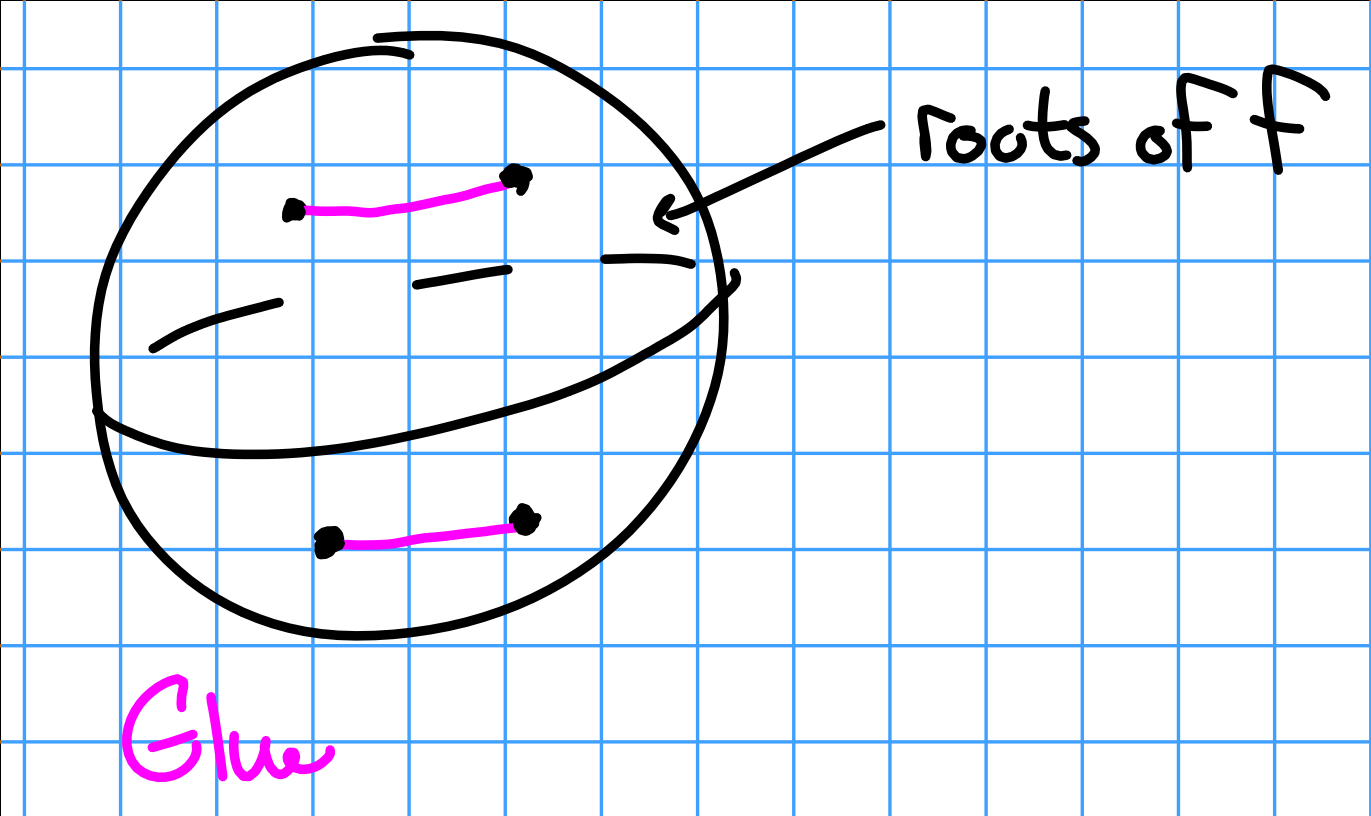Take $$E : y^2 = x^4 - 1$$, this is the only elliptic curve with an order 4 automorphism. In coordinates, this is generated by $$(x, y) \mapsto (-ix ,y)$$. So $$\omega = c \frac{dx}{y}$$, i.e. $$dx/y$$ up to scaling, and $$f^*(dx/y) = i \frac{dx}{y}$$. What is the constant $$c$$?

Take closed cycles on $$E$$ given by $$\alpha, \beta$$ (see diagram), then by FTC $$\int_\alpha \omega = z \mathrel{\Big|}_0^1 = 1$$. Negation is 4 fixed points on the elliptic curve, the 2-torsion points.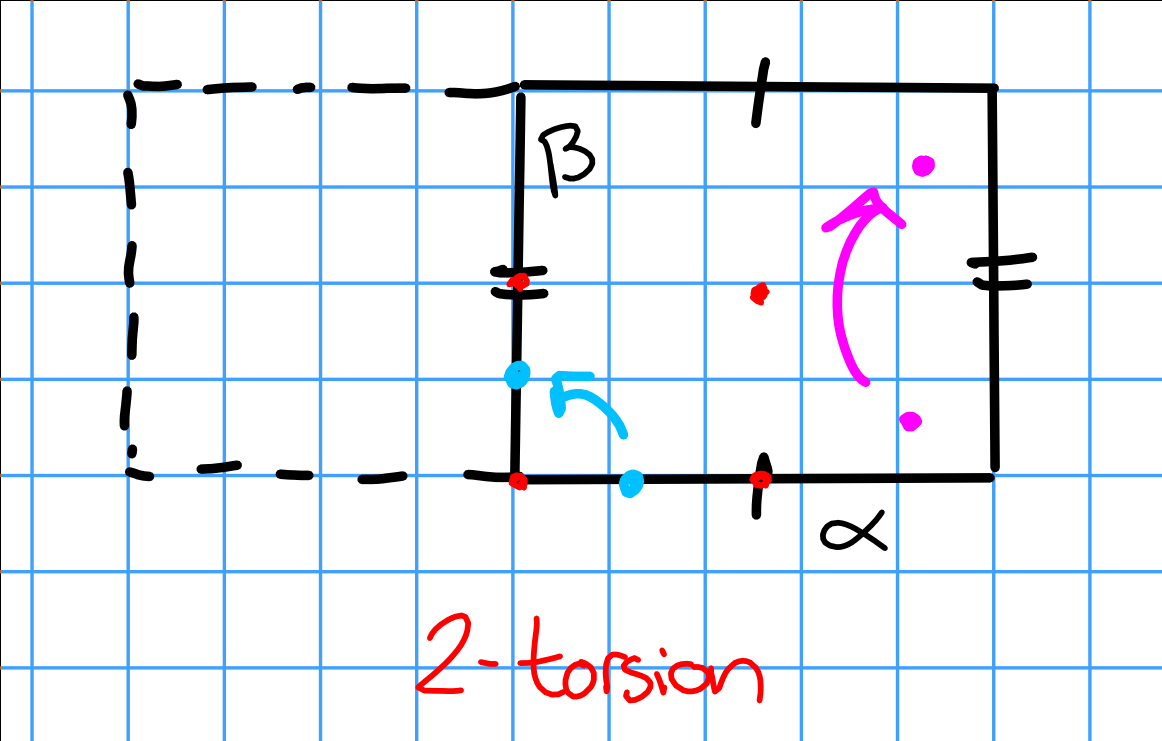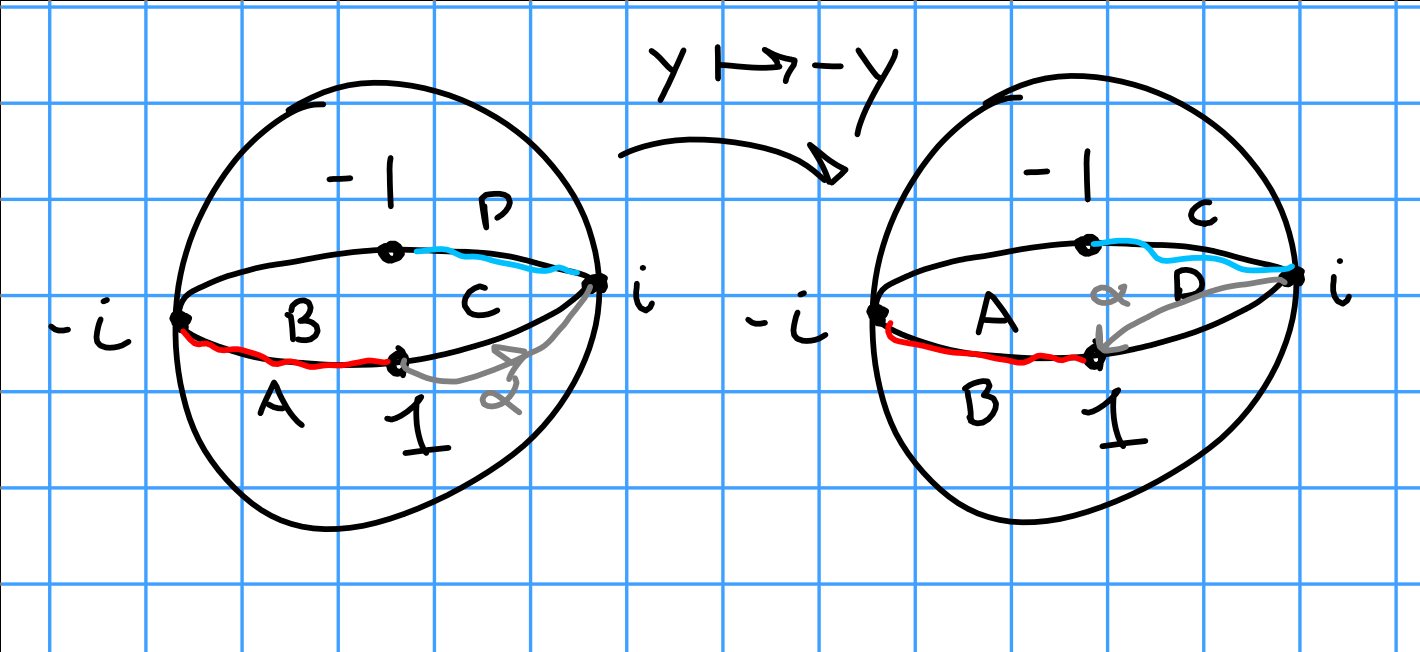We can then compute

\begin{align*} I = \int_\alpha \frac{dx}{y} = \int_1^2 \frac{dx}{\sqrt{x^4 - 1}} .\end{align*}

and since $$2c I = 1$$, this uniquely determines $$c$$.

Note that this can be numerically evaluated, but this is an elliptic integral with (possibly) no elementary antiderivative.

Consider the decagon with sides identified. We get a complex structure $$(\Sigma, \omega)$$ with cone angles $$4\pi$$ and $$dz$$ has 2 zeros of order 1 (namely the two cone points).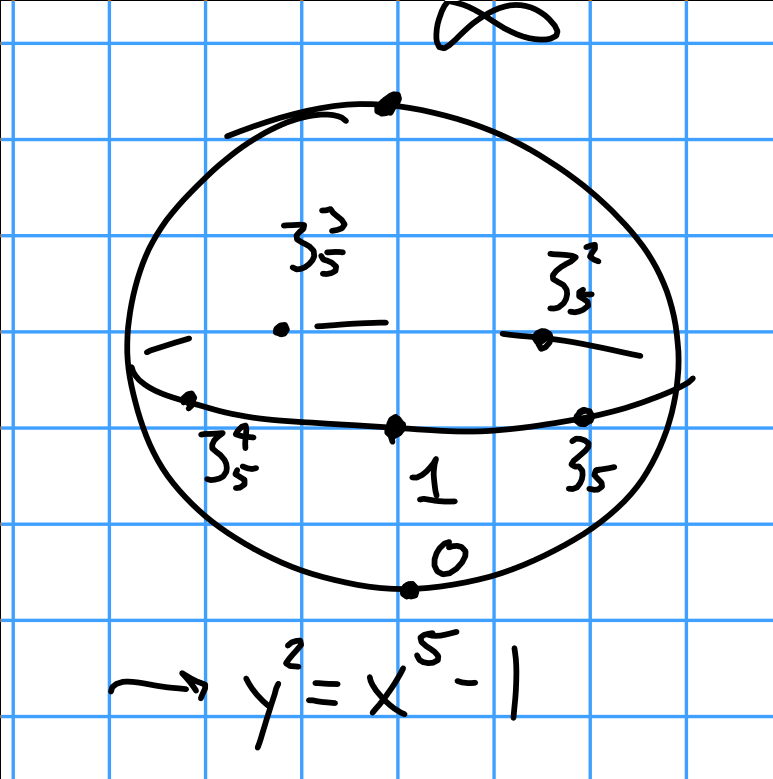What is the genus? The degree of the canonical bundle is \begin{align*} g(\Sigma) = \deg K_\Sigma = 2g - 2 = \sum_{p\in \Sigma}{\operatorname{Ord}}_p \omega \end{align*} and thus $$g = 2$$.

Fact: Every genus 2 curve is a double branched cover of $${\mathbb{P}}^1$$ branched over 6 points.

Use Riemann-Roch.

Consider automorphisms that preserve the decagon. Rotation by $$\pi/10$$ swaps the two cone points, to take rotation by $$-2\pi/5$$ (inserting the negative to account for pullbacks). Then $$f^* \omega = \zeta_5 \omega$$, where again we just write locally $$z \xrightarrow{f} \zeta_5^{-1}+ c \implies f^* dz = \zeta_5 dz$$.

Consider points of order $$5$$ on $${\mathbb{P}}^1$$, we can take $$\zeta_5^k$$ and $$\infty$$.This corresponds to the curves $$y^2 = x^5 - 1$$, with an automorphism $$(x, y) \mapsto (\zeta_5 x, y)$$. This is the only genus 2 curve with an order 5 automorphism.

Fact: The space of sections of the canonical $$H^0(K_{\mathcal E}) = {\mathbb{C}}^g$$.

We can write a basis for the space of 1-forms: $$\frac{dx}{y}$$ and $$(1-x) \frac{dx}{y}$$. Alternatively, $$\omega = (a+bx) \frac{dx}{y}$$ where $$a, b \in {\mathbb{C}}$$. What are the zeros of $$\omega$$? $$V(\omega) = V(a + bx)$$ where if $$b=0$$ it’s $$\infty$$. Because this has to preserve the order 5 symmetric and map cone points to themselves, this forces $$\omega = bx \frac{dx}{y}$$, which has exactly two zeros: $$(x, y) = (0, \pm i)$$.

We can also consider the doubled pentagon, which has only one point. This has an automorphism given by rotating each pentagon by $$1/5$$, it has cone angle $$6\pi$$, and $$\omega$$ has a double zero at the cone point. Since there is only one genus 2 curve with order 5 automorphisms, this yields the previous Riemann surface but a distinct translation surface and a distinct form.

We can write $$\alpha = a \frac{dx}{y}$$.

Proposition: One Riemann surface has many translation structures, and the space of such structures is the space of 1-forms.

Proof: Pick a chart $$w: U \to {\mathbb{C}}$$ avoiding the zeros of $$\omega$$. Then $$\omega = f(z) ~dz$$ in this chart, and we want to compose with a biholomorphism $$x$$ to obtain a new chart $$z$$ such that $$\omega = dz$$ in this chart. We can solve $$dz = e = f(w) ~dw$$ and $$\frac{dz}{dw} = f(w)$$ and by integrating we get $$z(w) = \int_{p_0}^w f(w_0) dw_0$$. In this chart, $$\omega = dz$$, and we can correct near zeros by finding charts such that $$\omega = z^n dz$$ where $$n$$ is the order of the zero.